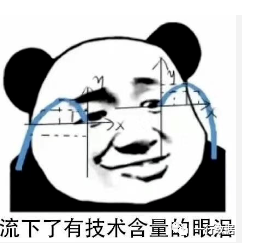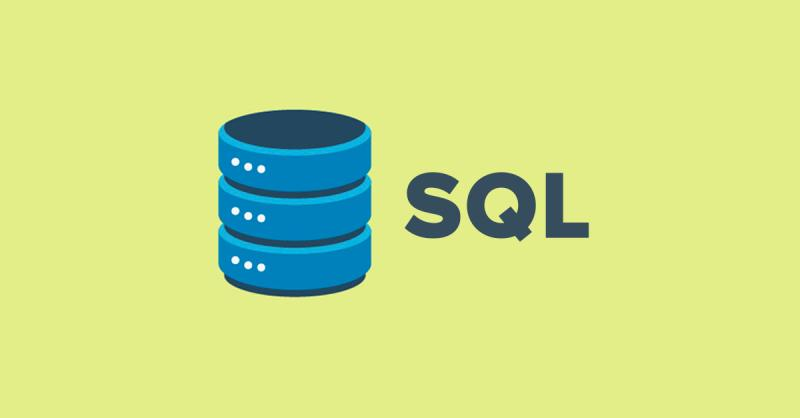+关注继续查看

# 引言和数据

pip install numpy

pip install scipy

pip install scikit-learn

pip install matplotlib

pip install pandas

pip install quandlimport pandas as pd
import quandl

df = quandl.get("WIKI/GOOGL")

print(df.head())

              Open    High     Low   Close    Volume  Ex-Dividend  \
Date
2004-08-19  100.00  104.06   95.96  100.34  44659000            0
2004-08-20  101.01  109.08  100.50  108.31  22834300            0
2004-08-23  110.75  113.48  109.05  109.40  18256100            0
2004-08-24  111.24  111.60  103.57  104.87  15247300            0
2004-08-25  104.96  108.00  103.88  106.00   9188600            0

Date
2004-08-19            1     50.000      52.03    47.980      50.170
2004-08-20            1     50.505      54.54    50.250      54.155
2004-08-23            1     55.375      56.74    54.525      54.700
2004-08-24            1     55.620      55.80    51.785      52.435
2004-08-25            1     52.480      54.00    51.940      53.000

Date
2004-08-19     44659000
2004-08-20     22834300
2004-08-23     18256100
2004-08-24     15247300
2004-08-25      9188600 

df = df[['Adj. Open',  'Adj. High',  'Adj. Low',  'Adj. Close', 'Adj. Volume']]

df['HL_PCT'] = (df['Adj. High'] - df['Adj. Low']) / df['Adj. Close'] * 100.0

df['PCT_change'] = (df['Adj. Close'] - df['Adj. Open']) / df['Adj. Open'] * 100.0

df = df[['Adj. Close', 'HL_PCT', 'PCT_change', 'Adj. Volume']]
print(df.head())
            Adj. Close    HL_PCT  PCT_change  Adj. Volume
Date
2004-08-19      50.170  8.072553    0.340000     44659000
2004-08-20      54.155  7.921706    7.227007     22834300
2004-08-23      54.700  4.049360   -1.218962     18256100
2004-08-24      52.435  7.657099   -5.726357     15247300
2004-08-25      53.000  3.886792    0.990854      9188600

# 特征和标签

import quandl
import pandas as pd

df = quandl.get("WIKI/GOOGL")
print(df.head())

import quandl, math
import numpy as np
import pandas as pd
from sklearn import preprocessing, cross_validation, svm
from sklearn.linear_model import LinearRegression

forecast_col = 'Adj. Close'
df.fillna(value=-99999, inplace=True)
forecast_out = int(math.ceil(0.01 * len(df)))

df['label'] = df[forecast_col].shift(-forecast_out)

# 训练和测试

import quandl, math
import numpy as np
import pandas as pd
from sklearn import preprocessing, cross_validation, svm
from sklearn.linear_model import LinearRegression

df = quandl.get("WIKI/GOOGL")

#print(df.tail())

df.fillna(value=-99999, inplace=True)
forecast_out = int(math.ceil(0.01 * len(df)))

df['label'] = df[forecast_col].shift(-forecast_out)

df.dropna(inplace=True)

X = np.array(df.drop(['label'], 1))
y = np.array(df['label'])

X = preprocessing.scale(X)

y = np.array(df['label'])

X_train, X_test, y_train, y_test = cross_validation.train_test_split(X, y, test_size=0.2)

clf = svm.SVR()

clf.fit(X_train, y_train)

confidence = clf.score(X_test, y_test)

print(confidence)
# 0.960075071072

clf = LinearRegression()
# 0.963311624499

clf = LinearRegression(n_jobs=-1)

for k in ['linear','poly','rbf','sigmoid']:
clf = svm.SVR(kernel=k)
clf.fit(X_train, y_train)
confidence = clf.score(X_test, y_test)
print(k,confidence)
linear 0.960075071072
poly 0.63712232551
rbf 0.802831714511
sigmoid -0.125347960903

# 预测

import quandl, math
import numpy as np
import pandas as pd
from sklearn import preprocessing, cross_validation, svm
from sklearn.linear_model import LinearRegression

df = quandl.get("WIKI/GOOGL")

df.fillna(value=-99999, inplace=True)
forecast_out = int(math.ceil(0.01 * len(df)))
df['label'] = df[forecast_col].shift(-forecast_out)

X = np.array(df.drop(['label'], 1))
X = preprocessing.scale(X)
X = X[:-forecast_out]
df.dropna(inplace=True)
y = np.array(df['label'])
X_train, X_test, y_train, y_test = cross_validation.train_test_split(X, y, test_size=0.2)

clf = LinearRegression(n_jobs=-1)
clf.fit(X_train, y_train)
confidence = clf.score(X_test, y_test)
print(confidence)

X = np.array(df.drop(['label'], 1))
X = preprocessing.scale(X)
X_lately = X[-forecast_out:]
X = X[:-forecast_out]

df.dropna(inplace=True)

y = np.array(df['label'])

X_train, X_test, y_train, y_test = cross_validation.train_test_split(X, y, test_size=0.2)
clf = LinearRegression(n_jobs=-1)
clf.fit(X_train, y_train)
confidence = clf.score(X_test, y_test)
print(confidence)

forecast_set = clf.predict(X_lately)

forecast_set是预测值的数组，表明你不仅仅可以做出单个预测，还可以一次性预测多个值。看看我们目前拥有什么：

[ 745.67829395  737.55633261  736.32921413  717.03929303  718.59047951
731.26376715  737.84381394  751.28161162  756.31775293  756.76751056
763.20185946  764.52651181  760.91320031  768.0072636   766.67038016
763.83749414  761.36173409  760.08514166  770.61581391  774.13939706
768.78733341  775.04458624  771.10782342  765.13955723  773.93369548
766.05507556  765.4984563   763.59630529  770.0057166   777.60915879] 0.956987938167 30

import datetime
import matplotlib.pyplot as plt
from matplotlib import style

style.use('ggplot')

df['Forecast'] = np.nan

last_date = df.iloc[-1].name
last_unix = last_date.timestamp()
one_day = 86400
next_unix = last_unix + one_day

for i in forecast_set:
next_date = datetime.datetime.fromtimestamp(next_unix)
next_unix += 86400
df.loc[next_date] = [np.nan for _ in range(len(df.columns)-1)]+[i]

df['Adj. Close'].plot()
df['Forecast'].plot()
plt.legend(loc=4)
plt.xlabel('Date')
plt.ylabel('Price')
plt.show()

import Quandl, math
import numpy as np
import pandas as pd
from sklearn import preprocessing, cross_validation, svm
from sklearn.linear_model import LinearRegression
import matplotlib.pyplot as plt
from matplotlib import style
import datetime

style.use('ggplot')

df = Quandl.get("WIKI/GOOGL")

df.fillna(value=-99999, inplace=True)
forecast_out = int(math.ceil(0.01 * len(df)))
df['label'] = df[forecast_col].shift(-forecast_out)

X = np.array(df.drop(['label'], 1))
X = preprocessing.scale(X)
X_lately = X[-forecast_out:]
X = X[:-forecast_out]

df.dropna(inplace=True)

y = np.array(df['label'])

X_train, X_test, y_train, y_test = cross_validation.train_test_split(X, y, test_size=0.2)
clf = LinearRegression(n_jobs=-1)
clf.fit(X_train, y_train)
confidence = clf.score(X_test, y_test)

forecast_set = clf.predict(X_lately)
df['Forecast'] = np.nan

last_date = df.iloc[-1].name
last_unix = last_date.timestamp()
one_day = 86400
next_unix = last_unix + one_day

for i in forecast_set:
next_date = datetime.datetime.fromtimestamp(next_unix)
next_unix += 86400
df.loc[next_date] = [np.nan for _ in range(len(df.columns)-1)]+[i]

df['Forecast'].plot()
plt.legend(loc=4)
plt.xlabel('Date')
plt.ylabel('Price')
plt.show()# 保存和扩展

import pickle

with open('linearregression.pickle','wb') as f:
pickle.dump(clf, f)

pickle_in = open('linearregression.pickle','rb')
clf = pickle.load(pickle_in)

import Quandl, math
import numpy as np
import pandas as pd
from sklearn import preprocessing, cross_validation, svm
from sklearn.linear_model import LinearRegression
import matplotlib.pyplot as plt
from matplotlib import style
import datetime
import pickle

style.use('ggplot')

df = Quandl.get("WIKI/GOOGL")

df.fillna(value=-99999, inplace=True)
forecast_out = int(math.ceil(0.1 * len(df)))

df['label'] = df[forecast_col].shift(-forecast_out)

X = np.array(df.drop(['label'], 1))
X = preprocessing.scale(X)
X_lately = X[-forecast_out:]
X = X[:-forecast_out]

df.dropna(inplace=True)

y = np.array(df['label'])

X_train, X_test, y_train, y_test = cross_validation.train_test_split(X, y, test_size=0.2)
#COMMENTED OUT:
##clf = svm.SVR(kernel='linear')
##clf.fit(X_train, y_train)
##confidence = clf.score(X_test, y_test)
##print(confidence)
pickle_in = open('linearregression.pickle','rb')

forecast_set = clf.predict(X_lately)
df['Forecast'] = np.nan

last_date = df.iloc[-1].name
last_unix = last_date.timestamp()
one_day = 86400
next_unix = last_unix + one_day

for i in forecast_set:
next_date = datetime.datetime.fromtimestamp(next_unix)
next_unix += 86400
df.loc[next_date] = [np.nan for _ in range(len(df.columns)-1)]+[i]
df['Forecast'].plot()
plt.legend(loc=4)
plt.xlabel('Date')
plt.ylabel('Price')
plt.show()# 理论以及工作原理

## 协方差# 编程计算斜率from statistics import mean
import numpy as np

xs = [1,2,3,4,5]
ys = [5,4,6,5,6]

xs = np.array([1,2,3,4,5], dtype=np.float64)
ys = np.array([5,4,6,5,6], dtype=np.float64)def best_fit_slope(xs,ys):
return m

m = best_fit_slope(xs,ys)

def best_fit_slope(xs,ys):
m = (mean(xs) * mean(ys))
return m

def best_fit_slope(xs,ys):
m = ( (mean(xs)*mean(ys)) - mean(xs*ys) )
return m

def best_fit_slope(xs,ys):
m = ( ((mean(xs)*mean(ys)) - mean(xs*ys)) /
(mean(xs)**2))
return m

def best_fit_slope(xs,ys):
m = (((mean(xs)*mean(ys)) - mean(xs*ys)) /
((mean(xs)**2) - mean(xs*xs)))
return m

from statistics import mean
import numpy as np

xs = np.array([1,2,3,4,5], dtype=np.float64)
ys = np.array([5,4,6,5,6], dtype=np.float64)

def best_fit_slope(xs,ys):
m = (((mean(xs)*mean(ys)) - mean(xs*ys)) /
((mean(xs)**2) - mean(xs**2)))
return m

m = best_fit_slope(xs,ys)
print(m)
# 0.3

# 计算纵截距

from statistics import mean
import numpy as np

xs = np.array([1,2,3,4,5], dtype=np.float64)
ys = np.array([5,4,6,5,6], dtype=np.float64)

def best_fit_slope(xs,ys):
m = (((mean(xs)*mean(ys)) - mean(xs*ys)) /
((mean(xs)*mean(xs)) - mean(xs*xs)))
return m

m = best_fit_slope(xs,ys)
print(m)def best_fit_slope_and_intercept(xs,ys):
m = (((mean(xs)*mean(ys)) - mean(xs*ys)) /
((mean(xs)*mean(xs)) - mean(xs*xs)))

b = mean(ys) - m*mean(xs)

return m, b

best_fit_slope_and_intercept(xs,ys)

from statistics import mean
import numpy as np

xs = np.array([1,2,3,4,5], dtype=np.float64)
ys = np.array([5,4,6,5,6], dtype=np.float64)

def best_fit_slope_and_intercept(xs,ys):
m = (((mean(xs)*mean(ys)) - mean(xs*ys)) /
((mean(xs)*mean(xs)) - mean(xs*xs)))

b = mean(ys) - m*mean(xs)

return m, b

m, b = best_fit_slope_and_intercept(xs,ys)

print(m,b)
# 0.3, 4.3regression_line = [(m*x)+b for x in xs]

regression_line = []
for x in xs:
regression_line.append((m*x)+b)

import matplotlib.pyplot as plt
from matplotlib import style
style.use('ggplot')

plt.scatter(xs,ys,color='#003F72')
plt.plot(xs, regression_line)
plt.show()predict_x = 7

predict_y = (m*predict_x)+b
print(predict_y)
# 6.4

predict_x = 7
predict_y = (m*predict_x)+b

plt.scatter(xs,ys,color='#003F72',label='data')
plt.plot(xs, regression_line, label='regression line')
plt.legend(loc=4)
plt.show()# R 平方和判定系数原理y_hat = x * m + b
r_sq = 1 - np.sum((y - y_hat) ** 2) / np.sum((y - y.mean()) ** 2)

# 编程计算 R 平方

def squared_error(ys_orig,ys_line):
return sum((ys_line - ys_orig) * (ys_line - ys_orig))

def coefficient_of_determination(ys_orig,ys_line):
y_mean_line = [mean(ys_orig) for y in ys_orig]
squared_error_regr = squared_error(ys_orig, ys_line)
squared_error_y_mean = squared_error(ys_orig, y_mean_line)
return 1 - (squared_error_regr/squared_error_y_mean)

from statistics import mean
import numpy as np
import matplotlib.pyplot as plt
from matplotlib import style
style.use('ggplot')

xs = np.array([1,2,3,4,5], dtype=np.float64)
ys = np.array([5,4,6,5,6], dtype=np.float64)

def best_fit_slope_and_intercept(xs,ys):
m = (((mean(xs)*mean(ys)) - mean(xs*ys)) /
((mean(xs)*mean(xs)) - mean(xs*xs)))
b = mean(ys) - m*mean(xs)
return m, b

def squared_error(ys_orig,ys_line):
return sum((ys_line - ys_orig) * (ys_line - ys_orig))

def coefficient_of_determination(ys_orig,ys_line):
y_mean_line = [mean(ys_orig) for y in ys_orig]
squared_error_regr = squared_error(ys_orig, ys_line)
squared_error_y_mean = squared_error(ys_orig, y_mean_line)
return 1 - (squared_error_regr/squared_error_y_mean)

m, b = best_fit_slope_and_intercept(xs,ys)
regression_line = [(m*x)+b for x in xs]

r_squared = coefficient_of_determination(ys,regression_line)
print(r_squared)
# 0.321428571429

##plt.scatter(xs,ys,color='#003F72',label='data')
##plt.plot(xs, regression_line, label='regression line')
##plt.legend(loc=4)
##plt.show()

# 为测试创建样例数据集

def create_dataset(hm,variance,step=2,correlation=False):

return np.array(xs, dtype=np.float64),np.array(ys,dtype=np.float64)

• hm（how much）：这是生成多少个数据点。例如我们可以选择 10，或者一千万。

• variance：决定每个数据点和之前的数据点相比，有多大变化。变化越大，就越不紧密。

• step：每个点距离均值有多远，默认为 2。

• correlation：可以为Falsepos或者neg，决定不相关、正相关和负相关。

def create_dataset(hm,variance,step=2,correlation=False):
val = 1
ys = []
for i in range(hm):
y = val + random.randrange(-variance,variance)
ys.append(y)

def create_dataset(hm,variance,step=2,correlation=False):
val = 1
ys = []
for i in range(hm):
y = val + random.randrange(-variance,variance)
ys.append(y)
if correlation and correlation == 'pos':
val+=step
elif correlation and correlation == 'neg':
val-=step

def create_dataset(hm,variance,step=2,correlation=False):
val = 1
ys = []
for i in range(hm):
y = val + random.randrange(-variance,variance)
ys.append(y)
if correlation and correlation == 'pos':
val+=step
elif correlation and correlation == 'neg':
val-=step

xs = [i for i in range(len(ys))]

return np.array(xs, dtype=np.float64),np.array(ys,dtype=np.float64)

xs, ys = create_dataset(40,40,2,correlation='pos')

from statistics import mean
import numpy as np
import random
import matplotlib.pyplot as plt
from matplotlib import style
style.use('ggplot')

def create_dataset(hm,variance,step=2,correlation=False):
val = 1
ys = []
for i in range(hm):
y = val + random.randrange(-variance,variance)
ys.append(y)
if correlation and correlation == 'pos':
val+=step
elif correlation and correlation == 'neg':
val-=step

xs = [i for i in range(len(ys))]

return np.array(xs, dtype=np.float64),np.array(ys,dtype=np.float64)

def best_fit_slope_and_intercept(xs,ys):
m = (((mean(xs)*mean(ys)) - mean(xs*ys)) /
((mean(xs)*mean(xs)) - mean(xs*xs)))

b = mean(ys) - m*mean(xs)

return m, b

def coefficient_of_determination(ys_orig,ys_line):
y_mean_line = [mean(ys_orig) for y in ys_orig]

squared_error_regr = sum((ys_line - ys_orig) * (ys_line - ys_orig))
squared_error_y_mean = sum((y_mean_line - ys_orig) * (y_mean_line - ys_orig))

print(squared_error_regr)
print(squared_error_y_mean)

r_squared = 1 - (squared_error_regr/squared_error_y_mean)

return r_squared

xs, ys = create_dataset(40,40,2,correlation='pos')
m, b = best_fit_slope_and_intercept(xs,ys)
regression_line = [(m*x)+b for x in xs]
r_squared = coefficient_of_determination(ys,regression_line)
print(r_squared)

plt.scatter(xs,ys,color='#003F72', label = 'data')
plt.plot(xs, regression_line, label = 'regression line')
plt.legend(loc=4)
plt.show()xs, ys = create_dataset(40,10,2,correlation='pos')xs, ys = create_dataset(40,10,2,correlation='neg')R 平方值是 0.930242442156，跟之前一样好，由于它们参数相同，只是方向不同。

xs, ys = create_dataset(40,10,2,correlation=False)Python学习十大公开免费数据集介绍

135 0116 0Python爬虫入门教程 31-100 36氪(36kr)数据抓取 scrapy
1. 36氪(36kr)数据----写在前面 今天抓取一个新闻媒体，36kr的文章内容，也是为后面的数据分析做相应的准备的，预计在12月底，爬虫大概写到50篇案例的时刻，将会迎来一个新的内容，系统的数据分析博文，记得关注哦~ 36kr 让一部分人先看到未来，而你今天要做的事情确实要抓取它的过去。
9517 0Python爬虫入门教程 28-100 虎嗅网文章数据抓取 pyspider
1. 虎嗅网文章数据----写在前面 今天继续使用pyspider爬取数据，很不幸，虎嗅资讯网被我选中了，网址为 https://www.huxiu.com/ 爬的就是它的资讯频道，本文章仅供学习交流使用，切勿用作其他用途。
1170 0Python零基础学习笔记（四）——数据类型
python数据类型：Number（数字）：包括整数/浮点数（小数）/复数String（字符串）Boolean（布尔值）None（空值）list（列表）tuple（元组）dict（字典）set（集合）
1278 0Python vs R : 在机器学习和数据分析领域中的对比
1123 0142 0ActiveReports 报表控件官方中文入门教程 (2)-创建、数据源、浏览以及发布

1062 0Python 学习笔记 - 列表，字符串，数据操作和字典
964 02914 0
apachecn_飞龙
Github：@wizardforcel 简书：@ApacheCN_飞龙 微博：@龙雀 CSDN：@wizardforcel ApacheCN 官网：apachecn.org 机器学习交流群：629470233
719

0

JS零基础入门教程（上册）